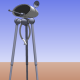## GIRC Path Data ConversionThe user inputs path data to GIRC by clicking points on the screen. Processing then draws segments between the points and calculates x,y coordinate data. This data is then converted into distance and turn data which will then be sent to the arduino aboard the robot.

In the above graphic, right turns are positive and left turns are negative. No turn can have a greater absolute value than 180. Turn calculations are rounded to the nearest degree. Initial heading is assumed to be zero degrees = positive x-axis.

What does this mean for the robot in practice? As an example, for the fourth and final path segment, the robot will be instructed to turn left 101 degrees, then travel 100 centimeters.

Now I can start work on sending the converted path data as commands to the robot over the USB/serial cable.

(NOTE: Data printed next to the points on the screen is for illustration purposes only. In real use, the data only appears in the lower left corner.)